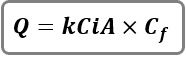# Rational Method Hydrographs

The Rational method was developed over 100 years ago and continues to be used for urban watershed modeling, typically on areas less than about 20 acres. Hydrology Studio can generate two kinds of Rational Method hydrographs;

1. Standard Rational
2. Modified Rational

## Standard Rational

The Standard Rational Method hydrograph is shaped like an isosceles triangle. The Peak is equivalent to the peak discharge as determined by the well known Rational formula.Where:
Qp = hydrograph peak discharge (cfs)
K = 1.0 (0.00278 metric)
C = runoff coefficient
A = basin area (ac)
i = intensity (in/hr) (Pulled from the IDF Curves. 5-minute minimum.)
Cf = frequency correction factor

The time-to-peak of the hydrograph is equal to the time of concentration. The ascending limb is equal to the time-to-Peak x (Ascending Limb Factor, ALF). The receding limb of the hydrograph is equal to the time-to-peak x (Receding Limb Factor, RLF). The hydrograph is an isosceles triangle when ALF = 1and RLF = 1. Intermediate hydrograph values are computed using straight-line interpolation.